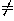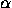Spherical Coordinates

# 9.5 Homogeneous Coordinates in Space

A quadruple of real numbers (x:y:z:t), with t0, is a set of homogeneous coordinates for the point P with cartesian coordinates (x/t, y/t, z/t). Thus the same point has many sets of homogeneous coordinates: (x:y:z:t) and (x':y':z':t') represent the same point if and only if there is some real numbersuch that x'=x, y'=y, z'=z, t'=t. If P has cartesian coordinates (x,y,z), one set of homogeneous coordinates for P is (x,y,z,1).

See Section 1.4 for more information on the relationship between cartesian and homogeneous coordinates. See Section 10.2 for formulas of space transformations in homogeneous coordinates.

Next: 10 Space Symmetries or Isometries
Up: 9 Coordinate Systems in Space
Previous: 9.4 Relations between Cartesian, Cylindrical, and Spherical CoordinatesThe Geometry Center Home Page

Silvio Levy
Wed Oct 4 16:41:25 PDT 1995

This document is excerpted from the 30th Edition of the CRC Standard Mathematical Tables and Formulas (CRC Press). Unauthorized duplication is forbidden.